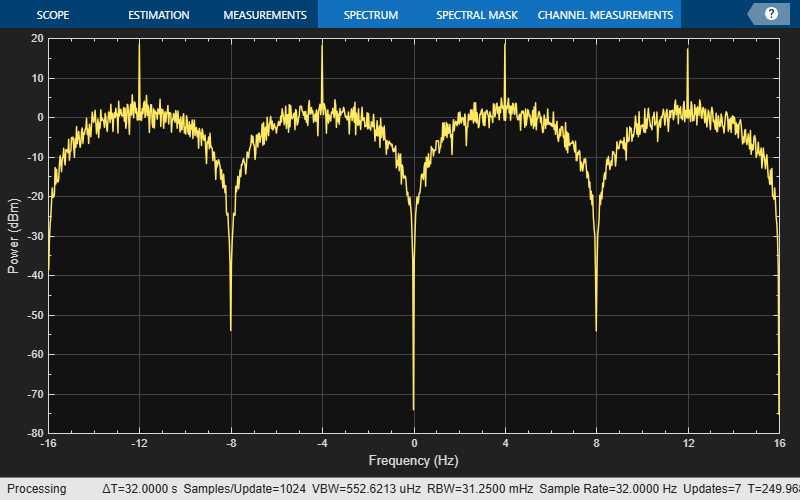# fskmod

Frequency shift keying modulation

## Syntax

``y = fskmod(x,M,freq_sep,nsamp)``
``y = fskmod(x,M,freq_sep,nsamp,Fs)``
``y = fskmod(x,M,freq_sep,nsamp,Fs,phase_cont)``
``y = fskmod(x,M,freq_sep,nsamp,Fs,phase_cont,symorder)``

## Description

````y = fskmod(x,M,freq_sep,nsamp)` outputs the complex envelope `y` of the modulation of the message signal `x` using frequency shift keying modulation.```
````y = fskmod(x,M,freq_sep,nsamp,Fs)` specifies the sampling rate of `y`.```

example

````y = fskmod(x,M,freq_sep,nsamp,Fs,phase_cont)` specifies the phase continuity.```
````y = fskmod(x,M,freq_sep,nsamp,Fs,phase_cont,symorder)` specifies how the function assigns binary words to corresponding integers.```

## Examples

collapse all

Generate an FSK modulated signal and display its spectral characteristics.

Set the function parameters.

```M = 4; % Modulation order freqsep = 8; % Frequency separation (Hz) nsamp = 8; % Number of samples per symbol Fs = 32; % Sample rate (Hz)```

Generate random M-ary symbols.

`x = randi([0 M-1],1000,1);`

Apply FSK modulation.

`y = fskmod(x,M,freqsep,nsamp,Fs);`

Create a spectrum analyzer System object™ and call it to display a plot of the signal spectrum.

```specAnal = spectrumAnalyzer(SampleRate=Fs); specAnal(y)```## Input Arguments

collapse all

Input signal, specified as a vector or matrix of positive integers. The elements of `x` must have values in the range of [0, `M` – 1]. If `x` is a matrix, `fskmod` processes the columns independently.

Example: `randi([0 3],100,1)`

Data Types: `double`

Modulation order, specified as an integer power of two.

Example: `2` | `4` | `16`

Data Types: `double`

Symbol order, specified as `'bin'` or `'gray'`. This argument specifies how the function assigns binary vectors to corresponding integers.

• If `symorder` is `'bin'`, the function uses binary-coded ordering.

• If `symorder` is `'gray'`, the function uses a Gray-coded ordering.

Data Types: `char`

Desired separation between frequencies, specified in Hz. By the Nyquist sampling theorem, `freq_sep` and `M` must satisfy (`M`-1)*`freq_sep` <= 1.

Data Types: `double`

Number of samples per output symbol, specified as a positive scalar greater than 1.

Data Types: `double`

Sample rate, specified as a positive scalar.

Data Types: `double`

Phase continuity, specified as either `'cont'` or `'discont'`. Set `phase_cont` to `'cont'` to force phase continuity across symbol boundaries in `y`, or `'discont'` to avoid forcing phase continuity.

Data Types: `char`

## Output Arguments

collapse all

Complex baseband representation of a FSK-modulated signal, returned as vector or matrix of complex values. The columns of `y` represent independent channels.

Data Types: `double` | `single`
Complex Number Support: Yes

 Sklar, Bernard. Digital Communications: Fundamentals and Applications. Upper Saddle River, NJ: Prentice-Hall, 2001.